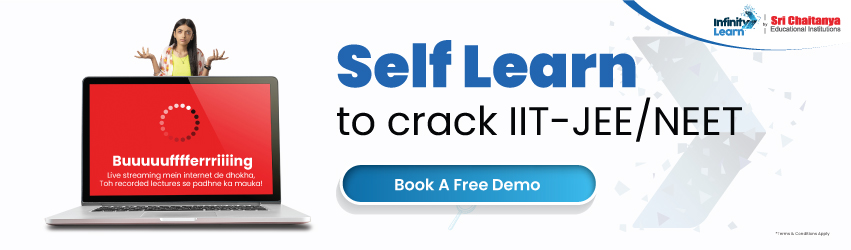NCERT Solutions for Class 12 Maths Chapter 7 Integrals (Ex 7.1) Exercise 7.1

# NCERT Solutions for Class 12 Maths Chapter 7 Integrals (Ex 7.1) Exercise 7.1infinitylearn surge self learn
infinitylearn starter package

Need FREE NCERT/CBSE/IIT-JEE/NEET Study Material?

Do you need help with your Homework? Are you preparing for Exams? Study without Internet (Offline)
×
Exercise 7.1

NCERT Solutions For Exercise 7.1 Maths Class 12

Introduction

Calculus is a discipline of mathematics that was developed independently in the latter part of the 17th century by Sir Isaac Newton and G.W Leibnitz (a German mathematician). The subject of calculus can be separated into two parts:

1. Calculus of Differences
2. Calculus of Integrals

Differential Calculus is concerned with determining the rate of change of a quantity such as a displacement, velocity, or acceleration, often known as finding the derivative of the quantity.

Integral Calculus aids in the determination of a quantity when the rate of change is known, implying that integration is the inverse of differentiation. This is why the anti-derivative of a function is called the integral of the function.

Integral Calculus Is Divided Into Two Types Once More.

1. Integral indefinite
2. Integral Definite

Definite Integral is used to discover the area limited by a curve given particular conditions, while Indefinite Integral is used to identify a function if its derivatives are known.

Note: Previously, calculating the area of basic forms with straight lines, such as a triangle, rectangle, or trapezium, was simple, but calculating the area under curved lines was difficult. This problem was solved with the use of integral calculus. Integral is widely used in a variety of domains, including engineering, physics, economics, and biology.

Infinity Learn presents several integration approaches that can assist you in solving the NCERT Solutions.

The following are the reasons why you should obtain the Class 12 Maths Chapter 7 Exercise 7.1 solution.

• Dispel Any Doubts

The first exercise does not need to be completed by the entire class. You can do it on your own and have reservations about it. After you’ve written down your questions, look at the Ex 7.1 Class 12 Maths NCERT Solutions to see how the experts came up with the solutions. Compare your results to those of the professionals to see where you may improve. As a result, you will be able to effortlessly clear your worries and progress to the next level without difficulty.

• At Its Finest, Convenience

There’s no need to be concerned when you have the Class 12 Chapter 7 Maths solution on hand. It is accessible offline and can be referred to at any time. Don’t waste time waiting for your mentors to come up with a solution. Take advantage of the answer and Learn how to tackle integral problems from the start.

• Creating A Foundation

As previously said, laying a foundation in integration concepts is essential for the future. You will not only do well on the board exam, but you will also pass the entrance exams. Use Exercise 7.1 Maths Class 12 Solutions to prepare your knowledge base for this.

FAQ:

1. Can you explain the distinction between definite and indefinite integrals?
2. What exactly do you mean when you say differential calculus?
3. How does Infinity Learn’s NCERT Solutions assist students to prepare for exams?

Q. Can you explain the distinction between definite and indefinite integrals?

Ans. The indefinite integral is used to identify a function if its derivative is known, whereas the definite integral is used to calculate the area circumscribed by a curve under particular conditions.

Q. What exactly do you mean when you say differential calculus?

Ans. Differential Calculus is concerned with determining the rate of change of a quantity such as a displacement, velocity, or acceleration, often known as finding the derivative of the quantity.

Q. How does Infinity Learn’s NCERT Solutions assist students to prepare for exams?

Ans. Infinity Learn’s NCERT Solutions will undoubtedly strengthen your analytical and mathematical abilities. For a better comprehension of the solutions, the solutions are presented step by step according to the CBSE board’s most recent syllabus. There are plenty of practice worksheets to aid you in your revision. Solving the NCERT Solutions will increase your self-assurance and help you prepare for the tests.

Join Infinity Learn Regular Class Programme!